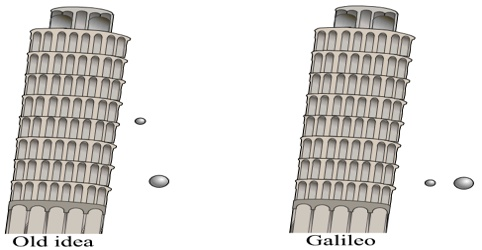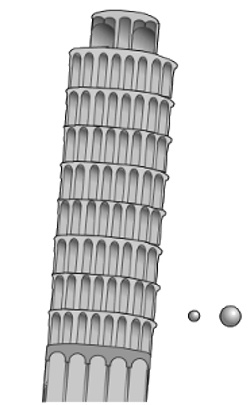# Laws of Falling Bodies

Laws of Falling Bodies

We know the reason behind the falling of the stone or paper is due to gravitational attraction. As acceleration due to gravity does not depend on mass then why the piece of paper and stone do not reach the ground at the same time? This unique property of gravity was one of the motivations for Einstein’s general theory of relativity. Galileo’s experiments with falling bodies; or investigations of falling bodies Galileo determined that the acceleration of these bodies is constant. Galileo Galili conducted extensive research on motion of falling bodies and gave some experimental laws. In 1589, he allowed to fall different heavy bodies from the top of an inclined pillar of 180 ft height and showed that these bodies fall on the ground almost at the same time [Figure].A small difference of delay in falling of the light and heavy bodies is due to air resistance. Later on Scientist Newton proved this law by the famous Guinea and Feather experiment Galileo gave three laws relating freely falling bodies like these. These laws are:

First law: In vacuum, all the freely falling bodies, starting from rest traverse equal distance in equal inters al of time. Or in vacuum all bodies starting from test tall with equal rapidity.

Explanation: Suppose two bodies having masses m1 and m2 fall downward from a long height without any hindrance and cover distances h1 and h2 in time t. Now according to this law, it will be found that h1 = h2.

Second law: Starting from rest, the velocity of a freely falling body is proportional to the time taken to fall.

Explanation: Suppose a body is falling to the ground from a great height under the action of gravitation force. In this case, if the velocity of the body is r. then v ∞ t.

or, v/t = constant; or, v1/t1 = v2/t2 = constant.

Here v1 and v2 are respectively the velocities in time t1 and t2.

Third law: Starting from rest, the distance traversed by a freely falling body is proportional to the square of the time to fall.

Explanation: Suppose a body is falling freely to the ground from a great height. If the distance traversed in time t is h, then according to this Law, h ∞ t2.

h/t2 = constant or h1/t12 = constant.

Here h1 and h2 are respectively the distance traversed at time t1 and t2.